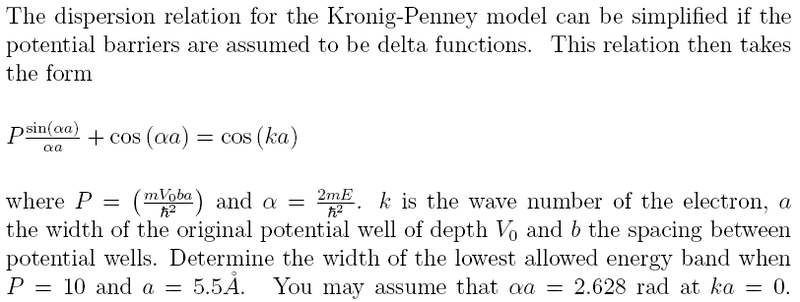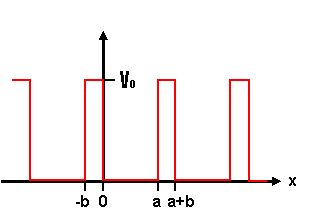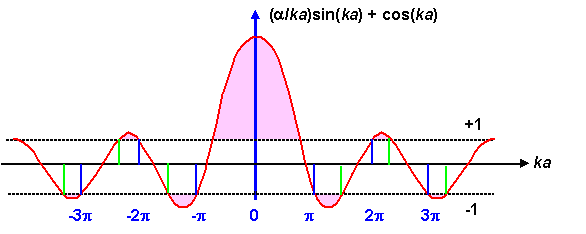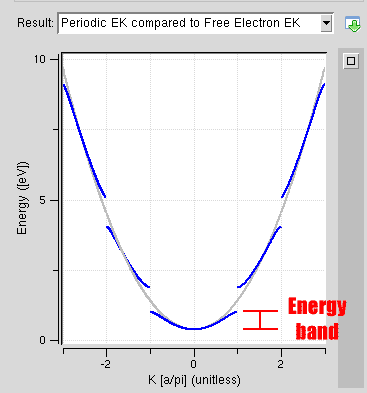# Energy bands from the Kronig-Penney model

mrneglect
I've got an exam tomorrow, and this is one of the topics I can't get my head around. Any help would be much appreciated.

## Homework Statement## The Attempt at a Solution

I've calculated that alpha=4.78x10^9. I understand that a Delta function is a periodic potential much like this one......EXCEPT that V0 --> infinity, b --> 0, but bV0 still has a finite area.

I can see that plotting the left-hand-side of the equation given in the question against (alpha x a) will result in this graph:And I understand that for the equation to hold true, the left-hand-side cannot go above 1 or below -1, hence the regions shaded pink in the above plot are invalid solutions of the equation in the question.

I understand that what I am looking for is the energy band marked here:But I just don't know how to get it.

I understand what the question is asking, what the concepts mean, but I'm just lost in the mathematics.

As I said, any help would be greatly appreciated.Cheers.

maverick280857
For the delta 'function' periodic potential, the Energy(E) versus Wavenumber(k) lies above the free particle parabola. So, the first band stretches from the global minimum of E(k) to the first intersection of E(k) with the parabola. You have all the information you need to work this out now.

This link might be useful to you:

http://webphysics.davidson.edu/faculty/thg/320_files/kronig-penney/kronig-penney.htm [Broken]

Specifically, refer to Figure 8.13(a).

Last edited by a moderator:
nagsper
i'm having similar problem but this time in plotting the figure 8.13(a). I'm using MatLab and I'm not getting it... is it possible to plot it without the eigenstates matrix? So I'm working with E versus (q*a) and I've searched for Matlab codes everywhere but all i got was more confused... i'v managed to plot the cos(q*a) versus (k*a) graphic (attachment) but not quite getting this one...